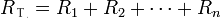# Calculating Resistors in Series

Resistors in Series calculations

The current through resistors in series stays the same, but the voltage across each resistor can be different. The sum of the potential differences (voltage) is equal to the total voltage.

The formula below will help you find the resistors in serias total resistance:R quals the sum of  R1 + R2 + R3 ....+ Rn

Example:  Assuming we have three resistors connected in series,
1k Ohm (1000 ohm), 820 Ohm and 1.5M Ohm (1,500,000 Ohm)

Then the total circuit resistence is 1,501,820 Ohms
(1000 Ohm + 820 Ohm + 1,500,000 Ohm = 1,501,820 Ohms)